# Analysis of Correlation Between the Price Of Leo token to that of Bitcoin 9-15th May, 2021The recent dip has called for concern to analyze data as regards bitcoin and leo token. This is an essential requirements to experimentally find the relationship between the two tokens.This guide covers the statistical analysis of random prices picked from coin gecko within a seven day range showing the price of leo token and that of bitcoin.

If you are a statistician, I would like you to also drop your comments or suggestion as regards for better improvement.

In the course of this article, I intend to cover the following namely: what correlation test is all about and theories of correlation, data collection and entry from coin gecko, data analysis and result interpretation using the spss software.

What Correlation Test Is All About
It’s been defined as a test that seeks to find the relationship between variables of concern. The variables we are concerned about is that of leofinance and that of bitcoin.

Sometimes when it is only two variables, It is referred to as bivariate correlation. Bivariate simply means there is a consideration of only two (bi) variables.
The theories attached to correlation analysis are;

Hypothesis
Hypothesis is a statement of fact of a data depending on the nature of test concerned about. The null hypothesis for correlation test is that there is no relationship between the variables in consideration.

Secondly, the correlation coefficient is determined by considering the following fact.

Negative correlation
A negative correlation between two variables indicates that the coefficient is negative as well and tends towards a negative one. Meaning that the higher one variable, the lower the other variable as well.

Positive correlation
A positive correlation indicates that the higher one variable, the higher the other variable. This entails the coefficient of correlation is positive as well and tends towards a positive one.

Zero correlation
Zero correlation indicates that there is no relationship at all between the variables in consideration and the correlation coefficient on a number line tends towards zero.

The coefficient of correlation resides on a line of -1 to 0 to +1.

The data below shows the price of leo token and bitcoin. It was entered using the variable and data view mode of spss.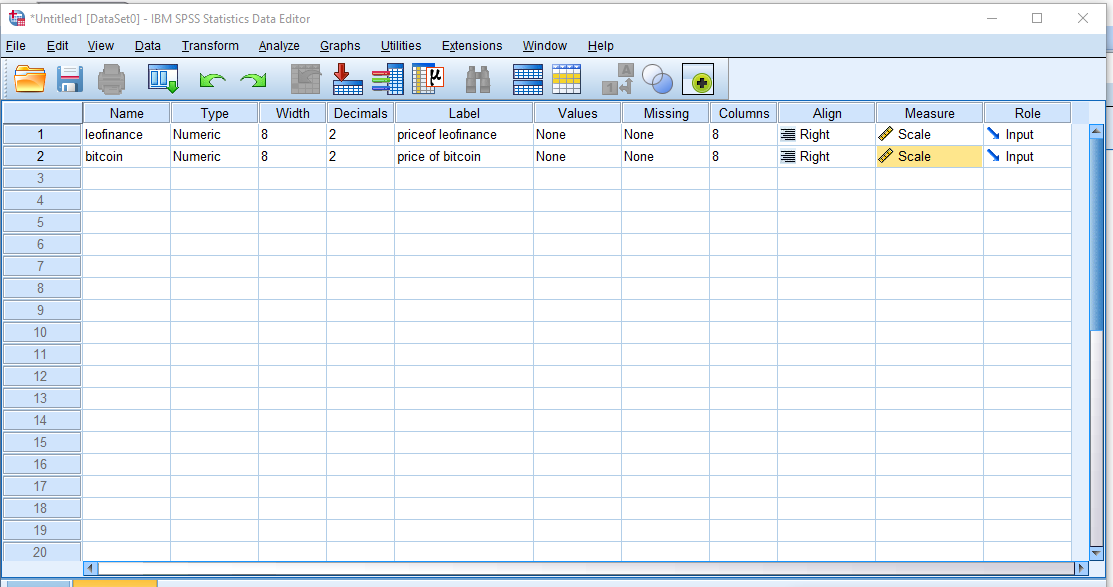Taken With Desktop

We shall be making use of 46 sample prices from coin gecko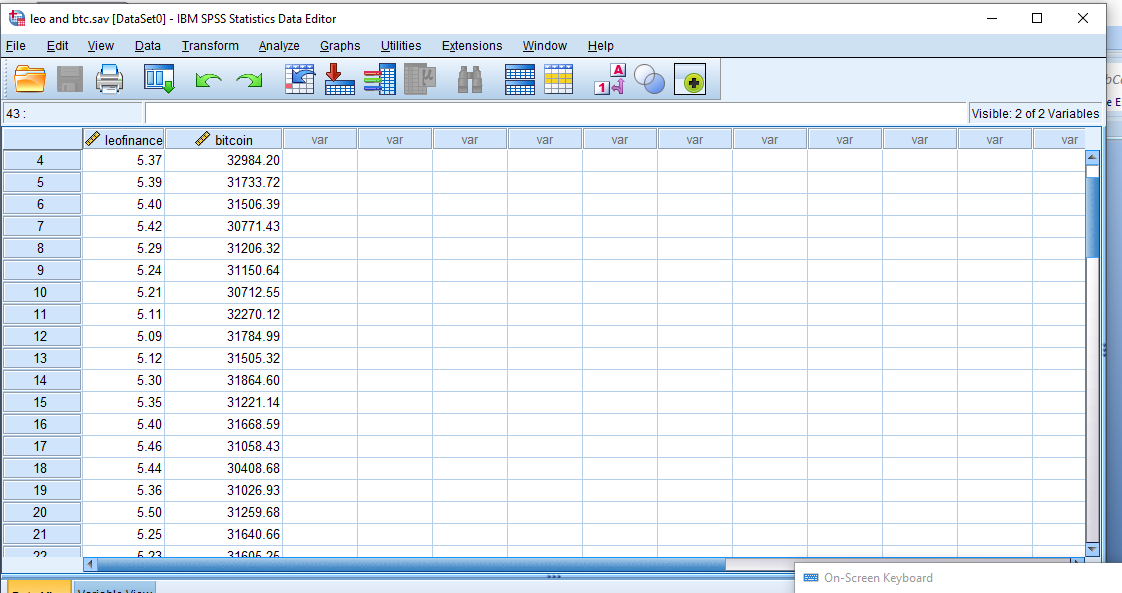Taken With Desktop

We shall intend to view the directionality of our data using scatter dot diagram as seen below.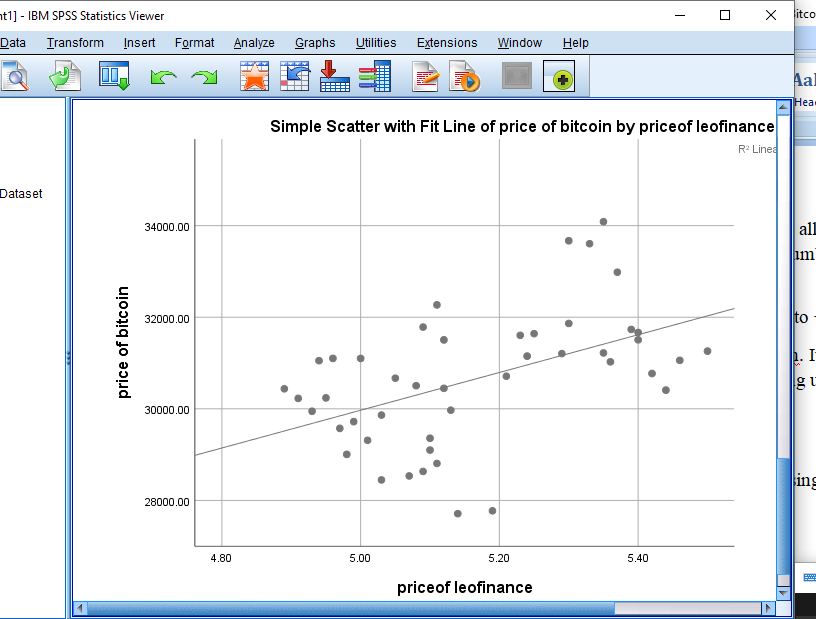Taken With Desktop

From our scatter plot, the direction of correlation tends to be a positive one.
Lets now proceed to our correlation analysis result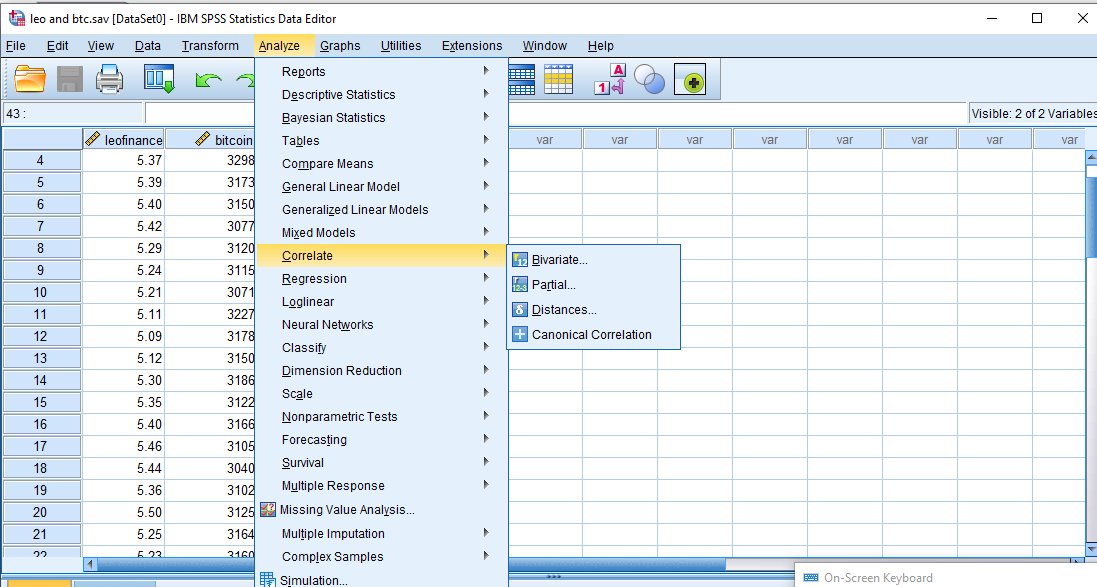Taken With Desktop

The result of the correlation analysis will measure the coefficient of correalion on a scale from -1 to 0 to +1.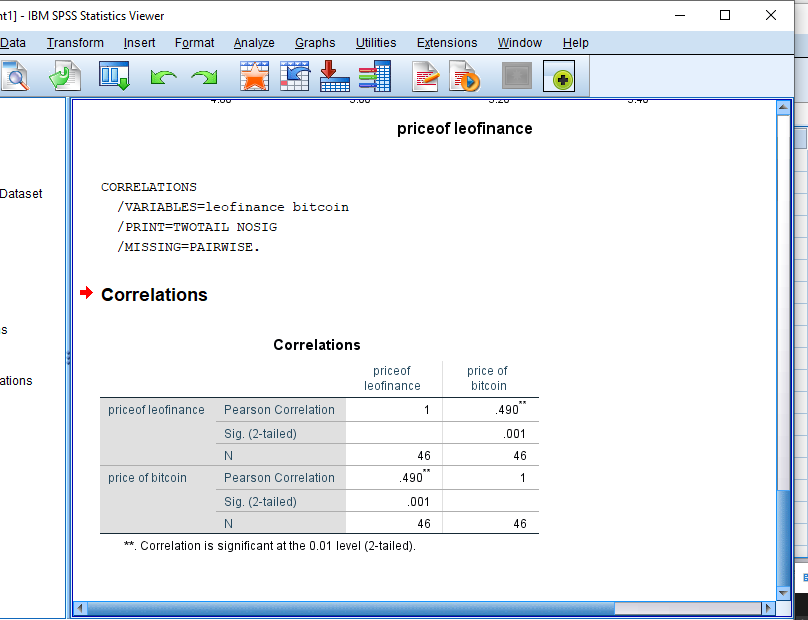Taken With Desktop

As said earlier, when it moves towards the positive one, the correlation coefficient is said to be interpreted as a postive one because the increase in one variable will lead to an increase in the other variable.

Interpretation of result
We compared to price in terms of relationship of leo token and bitcoin and there was proven to be a medium positive relationship between the prices of both coin meaning that when the price of bitcoin goes up there is a 50 – 50 chances that of leo goes up as well.

Conclusion
It has been nice playing with data from coin gecko as regards the price of leo token and that of bitcoin.

0
0
0.000